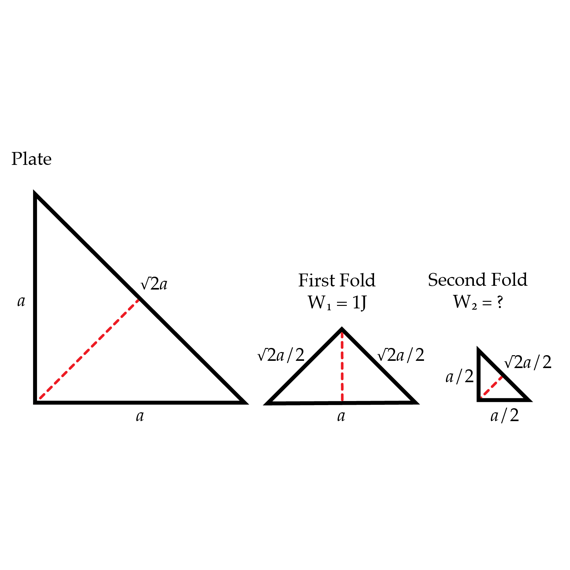# Folding a charged triangle.

A thin uniformly charged dielectric plate has the shape of a right isosceles triangle. The plate is folded to obtain a similar triangle. The work required to fold the plate is $W_{1}=1J$. What is the work in Joules $W_{2}$ required to fold it once more as in shown in the figure?×